Select Page

# Examples of DFA

By Making sure that you have already cover the topic of DFA. Now let see some examples of DFA in TOC.

## Example 1:

Design a DFA with ∑ = {0, 1} accepts the only input a string “10”.

### Solution:

In above example, the language contains only one string because it is specific in question

L= {10}

DFA for above language is given below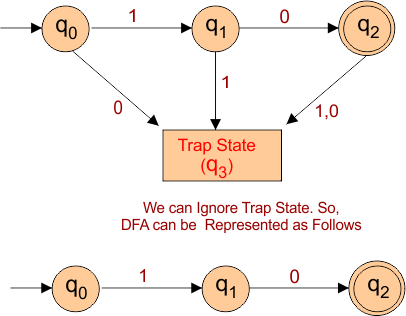## Example:2

Construct a DFA with ∑ = {a, b} accepts the only input “aaab”.

### Solution:

The language from given grammar is given below

L= {aaab}

DFA for above language is given below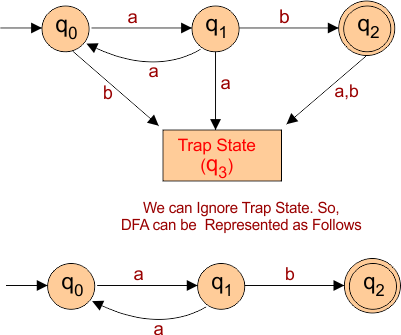## Example:3

Construct a DFA with sigma ∑ = {0, 1}, accepts those string which starts with 1 and ends with 0.

### Solution:

Language for given question is given below

L= {10, 1100,1010, 1110, 100010, 1000101110….}

Let draw the DFA which accept all the strings of above language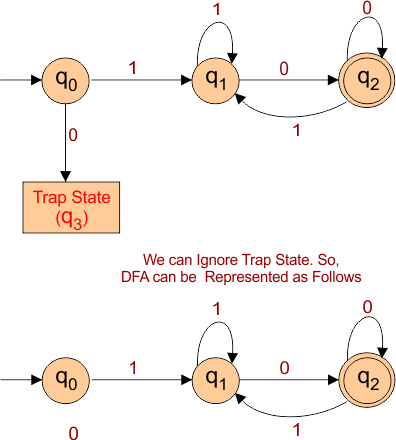Note: above automata machine does not accept those strings which are start and end with same input as “10001”

## Example:4

Construct a DFA with sigma ∑ = {0, 1}, accepts the set of all strings with three consecutive 0’s.

### Solution:

Language for given question is given below

L= {000, 1000,0001, 111000, 10001 ….}

Let draw the DFA which accept all the strings of above language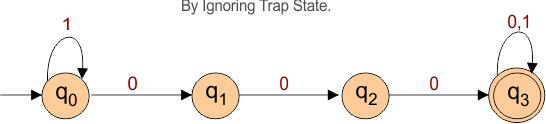## Example:5

Construct a DFA with sigma ∑ = {a, b}, accepts those strings which has even number of “a” and even number of “b”.

### Solution:

Language for given question is given below

L= { ε, aa, bb, abab, baab, aabb, aaaabbbb, bbbbaaaa, bbbbbbaaaaaa, ….}

Note: Epsilon (ε) represent zero which is even.

Let draw the DFA which accept all the strings of above language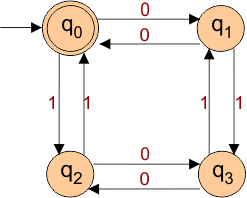In Above DFA,

• If state q1 becomes final instead of q0 then DFA will accept all those strings which have Odd “a” and even “b”.
• And if state q2 becomes final instead of q0 then DFA will accept all those strings which have even “a” and odd “b”.
• And If state q3 becomes final instead of q0 then DFA will accept all those strings which have odd “a” and odd “b”.

As given in following diagramHelp Other’s By Sharing…